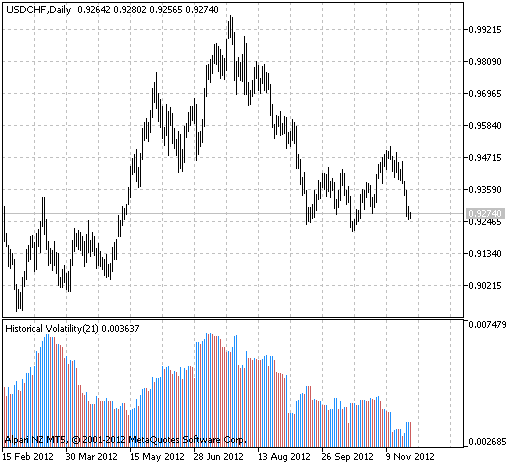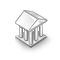Interesting script?
So post a link to it -
let others appraise it

You liked the script? Try it in the MetaTrader 5 terminal# i-VaR95 - indicator for MetaTrader 5

Views:
3429
Rating:
Published:
2012.12.25 11:31
Updated:
2016.11.22 07:32

The real author:

piccioli

The indicator displays the historical volatility value.

It is possible to calculate the volatility using several methods:

1. Simple Historical Volatility - a simple method of an equally weighed Moving Average;
2. Exponential Historical Volatility - a method of an exponential weighed Moving Average;
3. High-Low Historical Volatility - an extreme volatility, Parkinson's volatility.

Also there is possibility to calculate the risk costs with some probability.

This indicator was first implemented in MQL4 and published in Code Base at mql4.com on 19.03.2009.Fig.1 The i-VaR95 indicator

Translated from Russian by MetaQuotes Software Corp.
Original code: https://www.mql5.com/ru/code/1320Exp_MovingAverage_FN

The Expert Advisor is drawn on the basis of changing the direction of the MovingAverage_FN (digital hybrid and analog filtering indicator).RFractals

Fractals with the ability to set rangeATR_MA_Oscillator

ATR MA Oscillator is the oscillator drawn on the basis of the difference between the ATR oscillator and its signal line.PNN Neural Network Class

The class realizes the probabilistic neural network (Probabilistic Neural Network - PNN)